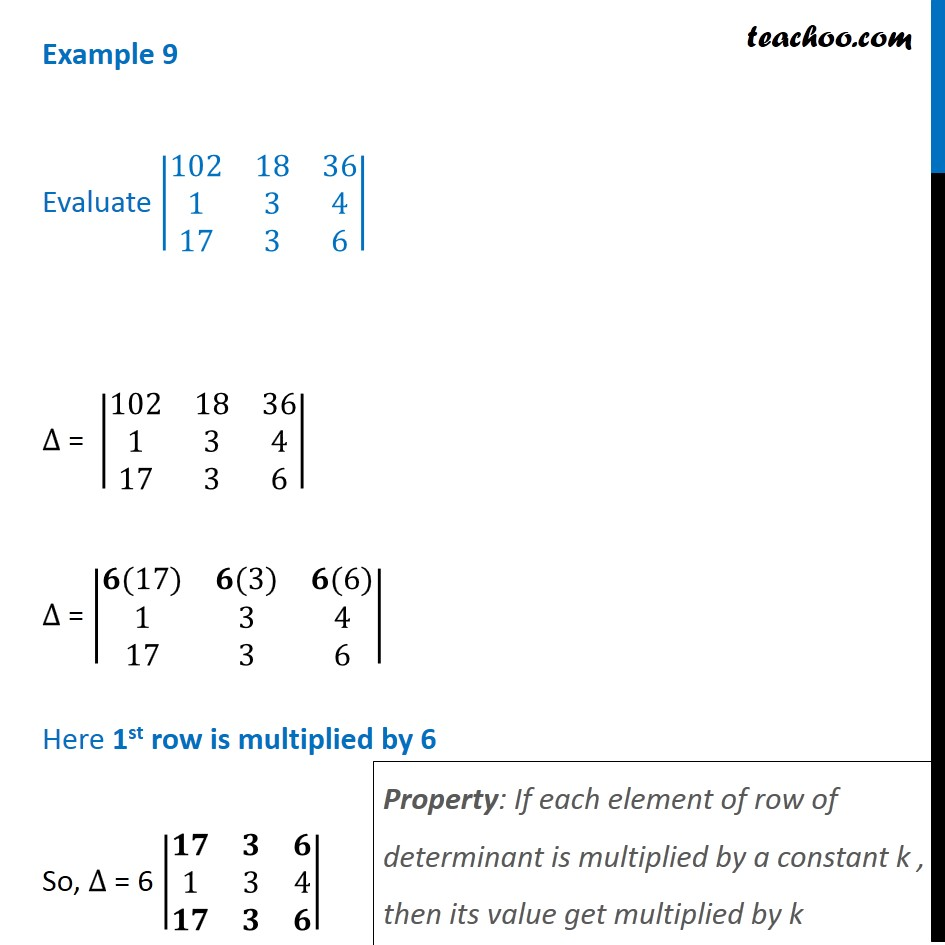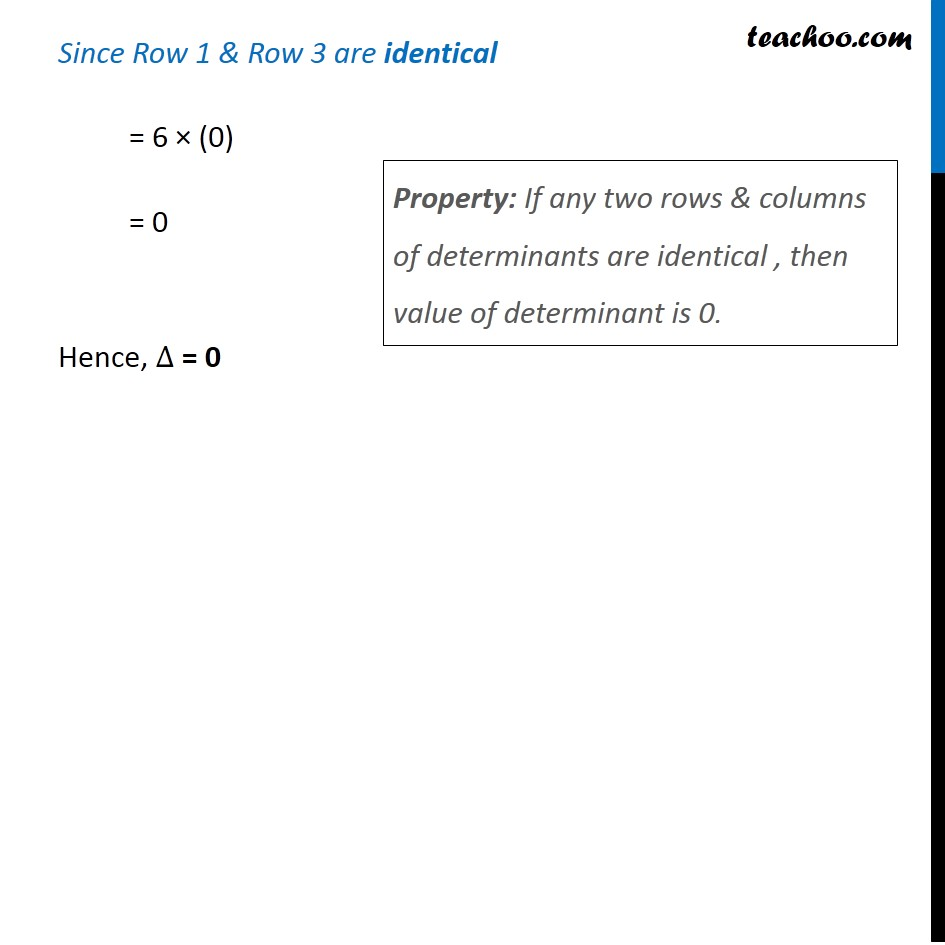1. Chapter 4 Class 12 Determinants (Term 1)
2. Serial order wise
3. Examples

Transcript

Example 9 Evaluate |■8(102&18&36@1&3&4@17&3&6)| ∆ = |■8(102&18&36@1&3&4@17&3&6)| ∆ = |■8(𝟔(17)&𝟔(3)&𝟔(6)@1&3&4@17&3&6)| Here 1st row is multiplied by 6 So, ∆ = 6 |■8(𝟏𝟕&𝟑&𝟔@1&3&4@𝟏𝟕&𝟑&𝟔)| Property: If each element of row of determinant is multiplied by a constant k , then its value get multiplied by k Since Row 1 & Row 3 are identical = 6 × (0) = 0 Hence, ∆ = 0 Property: If any two rows & columns of determinants are identical , then value of determinant is 0.

Examples